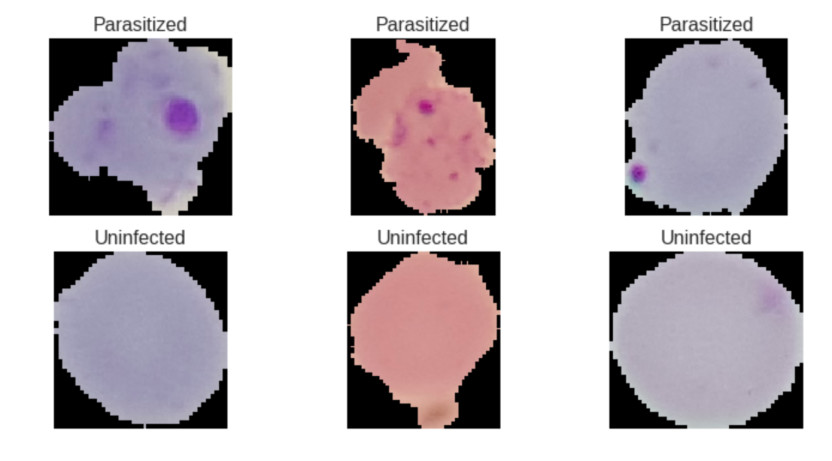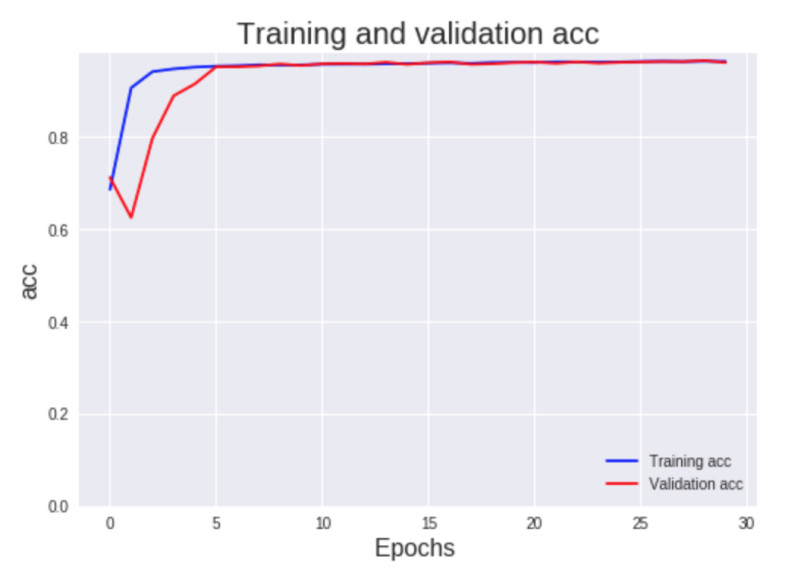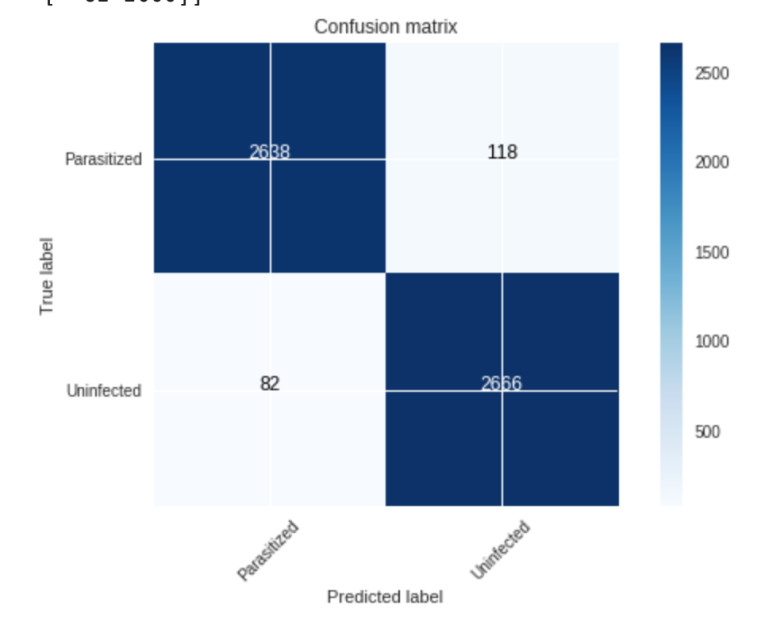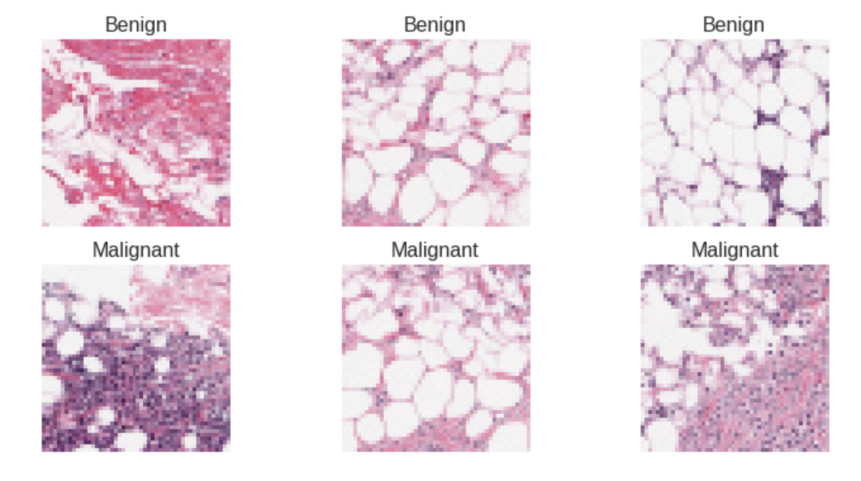April 24, 2019

Machine learning in healthcare part 2

Malaria

Here is the notebook with the code

Malaria is an infectious disease that involves fevers, shaking chills and anemia. This disease is caused by a parasite that is passed to humans by the bite of infected mosquitoes. The parasites enter the bloodstream and infect blood cells. Symptoms occur 10 days to 4 weeks after infection. More than 1 million people die of it every year.

Malaria can be tested by blood smears, a blood sample is taken from a patient and placed on a slide, clinicians examine the slide under a microscope and manually counts the infected blood cells, this process is time consuming and perfect for a convolutional neural network.

The dataset consists of 27,558 images, 13,779 for each class (Parasitized, Uninfected), due to the fact that the dataset is balanced it’s easer for the neural network to predict the correct class.

These images were obtained with a phone attached to a microscope.Mobile net architecture

I used the Mobile Net architecture since it works great on mobile devices like a cellphone.

Mobile net architecture uses layers called depthwise separable convolutions instead of normal convolutional layers, these layers separates the work in two operations: depthwise convolution and pointwise convolution. A normal convolutional layer has kernels to extract information from the image, if we have an input image of size 10x10x3 and one kernel of size 3x3x3 with a stride of 1 we end up with an output image of size 8x8x1.

Since we have an RGB image with 3 color channels (the depth), the kernel needs to have 3 channels as well, if we have 128 kernels, actually we could say that we have 384 kernels in total, 128 for each color channel. The output image will have a size of 8x8x128.

As I mentioned in the depthwise separable convolutions layers we separate the work into two operations, lets assume that we have the same input image of size 10x10x3 and we expect an output image of 8x8x128, first we compute the depthwise convolution where the output image will have a size of 8x8x3 since in this convolution we only have three kernels of size 3x3x1, one for each color channel and not 128, then we apply the pointwise convolution to this output image, this convolution uses a kernel of size 1x1x3 in order to remove the image’s depth and obtain and output image of size 8x8x1 then if we want an output image with a depth of 128 we use 128 kernels of size 1x1x3 to achieve this.

This could look weird and inefficient but if we do the maths:

In a normal convolutional layer if we have an input image of 10x10x3 and we want an output image of size 8x8x128 we need 128 kernels of size 3x3x3 that move 8x8 times:

128x3x3x3x8x8 = 221,184

A normal convolutional layer needs to do 221,184 operations to achieve this.

In a depthwise separable convolution layer in the first operation (depthwise convolution) we need 3 kernels of size 3x3x1 that move 8x8 times:

3x3x3x1x8x8 = 1,728

In the second operation (pointwise convolution) we need 128 kernels of size 1x1x3 that move 8x8 times:

128x1x1x3x8x8 = 24,576

A depthwise separable convolution layer only needs to do 26,304 operations whereas a normal convolutional layer needs 221,184.

Model

I used the Mobile Net v2 architecture with random weights, a learning rate of 0.0003 with decay and Stochastic gradient descent (SGD) as optimizer.

I trained the neural network for 30 epochs and a batch size of 32, the model reach an accuracy of 96.4% in the validation dataset. In this kind of problems is good to use metrics like sensitivity and specificity.In this model I obtained a sensitivity of 95.8% and a specificity of 97%.If the model has high sensitivity and it predicts a negative class (uninfected) we can be sure that the blood cell is uninfected whereas if the model has high specificity and it predicts a positive class (parasitized) we can be sure that the blood cell is parasitized.

I also made an iOS app that use this model with Tensorflow lite to detect parasitized or uninfected blood cells with the phone’s camera. You can see the code of this app on Github.

References

https://medlineplus.gov/ency/article/000621.htm

Breast cancer

Here is the notebook with the code

Breast cancer is the most common type of cancer in women, in this dataset we can find Invasive Ductal Carcinoma, the most common type of breast cancer.

This dataset contains 277,524 images of size 50x50 extracted from slide images,198,738 images belong to the class benign and 78,786 belongs to the class malignant, in this case we don’t have a balanced dataset.Malignant tumors spread to its surrounding tissues whereas benign tumors don’t invade its surrounding tissues.

Some methods to diagnose cancer can accelerate the spread of cancer to adjoining areas.

A secure method to detect signs of cancer is examine breast tissue from biopsies.

In this model I used almost the same parameters than the malaria model, Mobile Net v2 architecture with random weights, a learning rate of 0.0003 with decay and Stochastic gradient descent (SGD) as optimizer.I trained the model for 10 epochs with a batch size of 128, I obtain an accuracy of 86.8% in the validation set, a sensitivity of 85.6% and a specificity of 86%. I used class weights to balance the data, it means that the loss function will assign a higher importance to the malignant class that has fewer examples.